## Problem Solving Lesson 5: The perpendicular bisector of a line segment (C8 Math 7 Horizons) – Math Book

Solution for Exercise 5: The perpendicular bisector of a line segment (C8 Math 7 Horizon)
===========

### Solution 1 page 70 Math textbook 7 Creative horizon volume 2 – CTST

Figure 10 illustrates a piece of paper with a drawing of the median xy line of line segment AB, where the image of point B is blurred. Explain how point B is determined.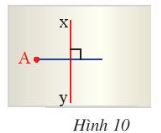Detailed instructions for solving Lesson 1

Solution method

– We find the intersection of the median and the line segment AB

– Then from that point find point B such that the distance from that point to A is equal to B and B, A and the intersection must be collinear, B does not coincide with A

Detailed explanation

Let the intersection of AB and xy be O .

\( \Rightarrow \) O is the midpoint of AB ( Do xy is the perpendicular bisector of AB)

\( \Rightarrow \) Measure the distance AO and from point O draw OB such that OA = OB and lies on the opposite side of point A from the line xy ( A, B, O align )

–>

— *****

### Solution 2 page 70 Math textbook 7 Creative horizon volume 2 – CTST

Observe Figure 11, show that M is the midpoint of BC, AM is perpendicular to BC and AB = 10 cm, Calculate AC.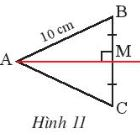Detailed instructions for solving Lesson 2

Solution method

We prove that the two triangles AMC and AMB are congruent

Detailed explanation

Consider triangle AMB and triangle AMC with:

AM common edge

MB = MC (since M is the midpoint of BC)

\(\widehat {BMA} = \widehat {CMA} = {90^o}\)

\( \Rightarrow \) Triangle AMB = Triangle AMC (cgc)

\( \Rightarrow \)AB = AC = 10 cm (corresponding sides are equal)

–>

— *****

### Solution 3 page 70 Math textbook 7 Creative horizon volume 2 – CTST

Observe Figure 12, show that AM is the perpendicular bisector of line segment BC and DB = DC = 8 cm. Prove that the three points A, M, D are collinear.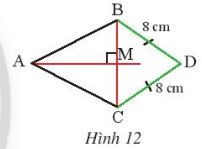Detailed instructions for solving Lesson 3

Solution method

Prove that D belongs to the perpendicular bisector of BC \( \Rightarrow \) A, M, D are collinear

Detailed explanation

Consider triangle BCD with BD = CD (assumption )

\( \Rightarrow \) D belongs to the perpendicular bisector of BC because it is equidistant from the two ends of segment BC

Where AM is the perpendicular bisector of BC

\( \Rightarrow \) D belongs to the line AM

\( \Rightarrow \) A, M, D align

–>

— *****

### Solution 4 page 70 Math textbook 7 Creative horizon volume 2 – CTST

Observe Figure 13, know AB = AC, DB = DC. Prove that M is the midpoint of BC.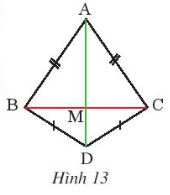Detailed instructions for solving Lesson 4

Solution method

– We prove that the two triangles ABD and ACD are congruent

– Infer 2 triangles BAM and CAM are equal \( \Rightarrow \) BM = CM

Detailed explanation

Consider triangle ABD and triangle ACD with:

AB = AC (assumption )

BD = CD (assumption)

\( \Rightarrow \Delta ABD =\Delta ACD (ccc)\)

Consider triangle ABM and triangle ACM:

AB = AC (assumption )

AM common edge

\(\Delta ABM=\Delta ACM (cgc)\)

\(\Rightarrow MC = MB\) ( 2 corresponding edges )

\( \Rightarrow \) M is the midpoint of BC

–>

— *****

### Solve problems 5 pages 70 Math textbook 7 Creative horizon volume 2 – CTST

Let two points M and N lie on the perpendicular bisector d of the line segment EF. Prove that \(\Delta EMN=\Delta FMN\)

Detailed instructions for solving Lesson 5

Solution method

Prove that 2 triangles are congruent by case (ccc)

Detailed explanationSince M belongs to the orthogonal EF, ME = MF (property of the point on the orthogonal )

Similarly \( \Rightarrow \) NE = NF (the property of the point on the orthogonal )

Consider two triangles MEN and MFN with :

MN is a common edge

ME = MF

NE = NF

\(\Rightarrow \Delta MEN = \Delta MFN (ccc)\)

–>

— *****

### Solve problem 6 page 70 Math textbook 7 Creative horizon volume 2 – CTST

On the planning map of a residential area, there is a road d and two residential points A and B (Figure 14). Find on the roadside a location M to build a medical station such that the medical station is equidistant from two residential points.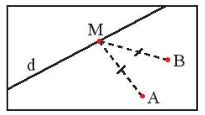Detailed instructions for solving Lesson 6

Solution method

– Let MA = MB \( \Rightarrow \) M belongs to the orthogonal AB

– Find M in d

Detailed explanation

Let M be equidistant from A, B if and only if M is on the perpendicular bisector of AB

Since M must belong to d \( \Rightarrow \) M is the intersection of the perpendicular bisector AB and the line d

–>

— *****